# What is the compressibility for a Vanderwall's gas at its Boyle's Temp?What's the approach used?

12 years ago

TB can be derived from the va n der Waals equation of state for a real gas,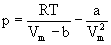In order to do that, we express the van der Waals equation as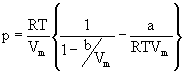So long as b/Vm < 1, we can expand the first term inside the brackets according to

(1 – x)-1 = 1 + x + x2 + ...

which gives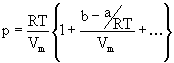Obviously the virial coefficient B is given by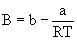At the Boyle Temperature B = 0, and so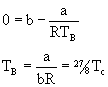(Tc = 8a/27Rb is the critical temperature of the gas.)

Thus, the Boyle temperature may be found for any gas for which the critical temperature has been determined.

12 years ago

So does that mean its 1 for low pressures and greater than 1 for high pressures ?